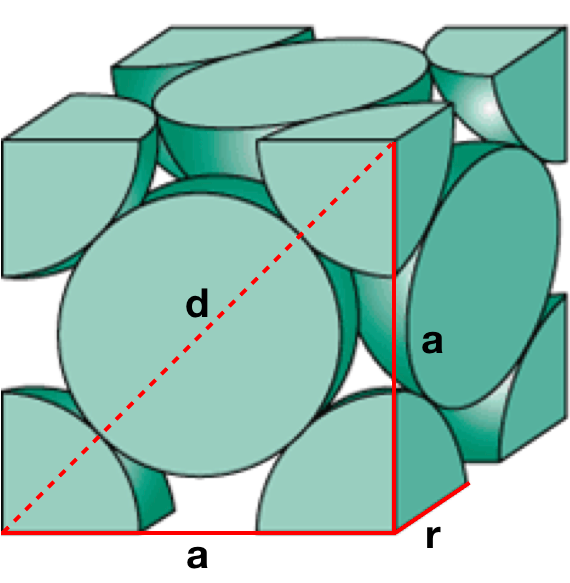# Problem: Copper crystallizes in a face-centered cubic lattice. If the edge of the unit cell is 351 pm, what is the radius of the copper atom?

95% (92 ratings)
###### FREE Expert Solution

We’re being asked to determine the radius of a copper atom knowing that it follows a face-centered cubic lattice with an edge length of 351 pm.

Recall that the face-centered cubic lattice contains an atom in each of the face of the cube and an atom in each of the corners. It looks like this:95% (92 ratings)###### Problem Details

Copper crystallizes in a face-centered cubic lattice. If the edge of the unit cell is 351 pm, what is the radius of the copper atom?

What scientific concept do you need to know in order to solve this problem?

Our tutors have indicated that to solve this problem you will need to apply the Unit Cell concept. You can view video lessons to learn Unit Cell. Or if you need more Unit Cell practice, you can also practice Unit Cell practice problems.

What is the difficulty of this problem?

Our tutors rated the difficulty ofCopper crystallizes in a face-centered cubic lattice. If the...as medium difficulty.

How long does this problem take to solve?

Our expert Chemistry tutor, Sabrina took 1 minute and 34 seconds to solve this problem. You can follow their steps in the video explanation above.

What professor is this problem relevant for?

Based on our data, we think this problem is relevant for Professor Esfandiari's class at SJSU.